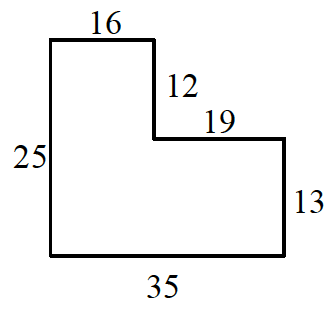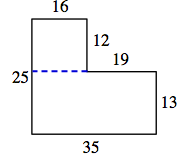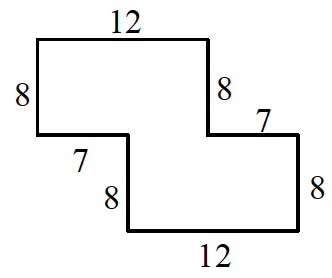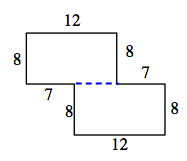### Home > AC > Chapter 2 > Lesson 2.1.1 > Problem2-8

2-8.

Copy the following figures onto your paper. Then find the area and perimeter of each shape. Assume that all corners are right angles. Show all work.  Homework Help ✎

1.To find the area, separate the shape into two boxes, find the area of the two sections, and then add them together for the total area.

$16\times12=192$
$13 \times 35 = 455$
area $=192+455$

area $=647$ units2

To find the perimeter (the distance around a shape) add up all of the sides of the shape.

perimeter $=16+12+19+13+35+25$

perimeter $=120$ units

1.To find the area and perimeter of the shape in part (b), follow the same strategy outlined in part (a).